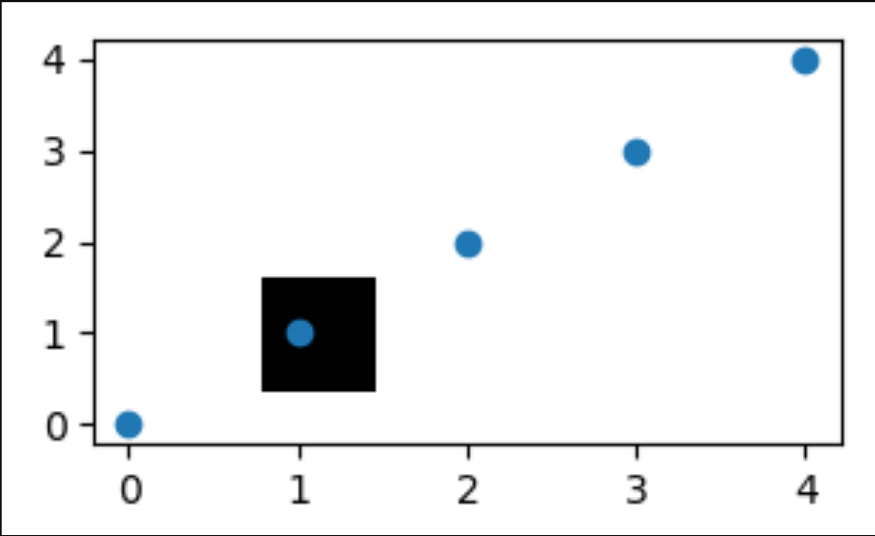Matplotlib.pyplot.figimage() function in Python

• Last Updated : 10 Jul, 2020

Matplotlib is a widely used library in Python for plotting various graphs, as it provides very efficient ways and easy to understand methods for complex plots also. pyplot is a collection of command style functions that make matplotlib work like MATLAB.

figimage() function

matplotlib.pyplot.figimage() is a method for adding a non-resampled image to the figure. Usually, it attaches the image to the lower or upper left corner depending on origin.

Syntax: matplotlib.pyplot.figimage(*args, **kwargs)

Parameters:

alpha is a float valued parameter, It is used to give the blending value for the image. It is an optional parameter. When not specified, it takes the default value as ‘None’.

Xo, Yo is another important parameter which states the shape of the image data. Usually it can be ‘both’, ‘x’, or ‘y’. It takes the x/y image offset in pixels.

**kwargs It is an additional parameter generally known as kwargs. They are Artist kwargs passed on to FigureImage.

Now let us take a simple examples to illustrate the working of matplotlib.pyplot.figimage().

Example 1:

 import numpy as npimport matplotlibimport matplotlib.pyplot as plt    fig = plt.figure()Z = np.arange(10000).reshape((100, 100))Z[:, 50:] = 1  im1 = fig.figimage(Z, xo = 50, yo = 0,                   origin ='lower')im2 = fig.figimage(Z, xo = 100, yo = 100,                   alpha =.8, origin ='lower')  plt.show()

Output:Example 2:

 import numpy as npimport matplotlib.pyplot as plt  im = np.zeros((40, 40, 3), dtype = np.float)  fig, ax = plt.subplots()im = fig.figimage(im, 100, 60)  ax.scatter([0, 1, 2, 3, 4], [0, 1, 2, 3, 4])  ax.set_zorder(1)im.set_zorder(0)ax.patch.set_visible(False)  plt.show()

Output:Attention geek! Strengthen your foundations with the Python Programming Foundation Course and learn the basics.

To begin with, your interview preparations Enhance your Data Structures concepts with the Python DS Course. And to begin with your Machine Learning Journey, join the Machine Learning - Basic Level Course

My Personal Notes arrow_drop_up